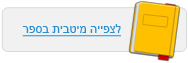עמוד:38 Summary Questions 1 . What types of energy are manifested in the events listed in the table ? Indicate “ + ” in the correct cells . 2 . Complete : A skateboarder is traveling down a slope . During his downward travel , his __________ energy turns into ___________ energy . At the same time , due to the friction between the skateboard wheels and the slope , and the axles supporting them , as well as the friction between the skateboarder and the air , the skateboarder’s __________ energy is converted to __________ . 3 . Draw energy flow charts depicting the transformations of energy in the following examples : a . An electric heater heats up by means of electrical energy . b . A bus uses fuel to travel on the road . c . A hydroelectric power plant uses a waterfall to obtain electrical energy . The electrical energy is used to power an electric light bulb in a home . d . A child falls onto a trampoline , hits it , and bounces back up . 4 . What kind of energy change occurs in each of the events listed in the following table ? Indicate “ + ” in the correct cells ( only once in each row ) . 5 . What is the law of conservation of energy ? a . A law aimed at preventing unnecessary use of fuels , designed to enable savings in energy and prevent air pollution . b . Energy may change its form and be transferred from one body to another ; however , in the case of a closed system the total amount of energy remains constant . c . A recommendation to conserve energy for a future hour of need . d . A law saying that it is impossible to fully utilize energy . 6 . A basketball is bounced from the floor upwards . What happens from the moment the ball bounces up until the moment it hits the floor again , assuming there is no friction . a . The sum total of the ball’s kinetic energy and potential energy decreases while it is rising . b . The sum total of the ball’s kinetic energy and potential energy increases while it is rising . c . The sum total of the ball’s kinetic energy and potential energy remains constant . d . The sum total of the ball’s kinetic energy and potential energy changes according to the circumstances .© מטח - המרכז לטכנולוגיה חינוכית